Next: Current Divider Up: Kirchoff's Laws Previous: Series and Parallel Combinations

## Voltage Divider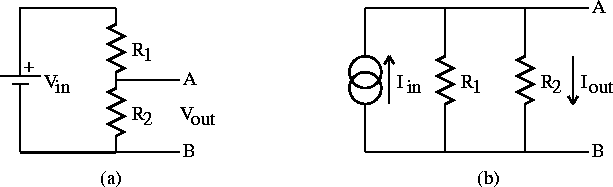Figure 1.3:   Divider circuits: a) voltage divider and b) current divider.

Consider the voltage divider shown in figure 1.3a. The voltage across the input source is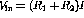and the voltage across the output between terminals A and B is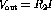. The output voltage from the voltage divider in thus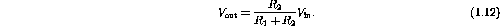Example: Determine an expression for the voltage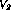on the voltage divider in figure 1.4.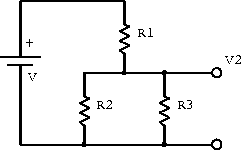Figure 1.4:  Example voltage divider.

We take the bottom line in figure 1.4 to be at ground and define the current flowing betweenand ground to be I. Ohm's law gives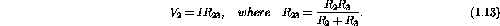Applying Kirchoff's voltage law for the input source gives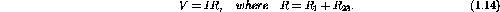Combining the above two results and solving forleads to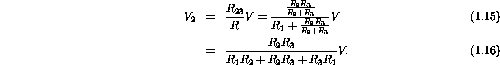Doug Gingrich
Tue Jul 13 16:55:15 EDT 1999